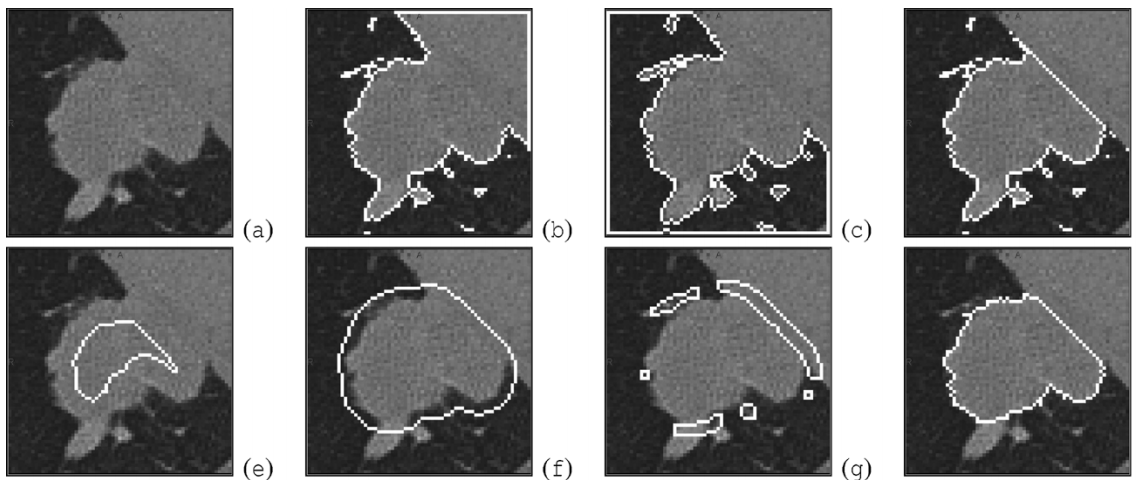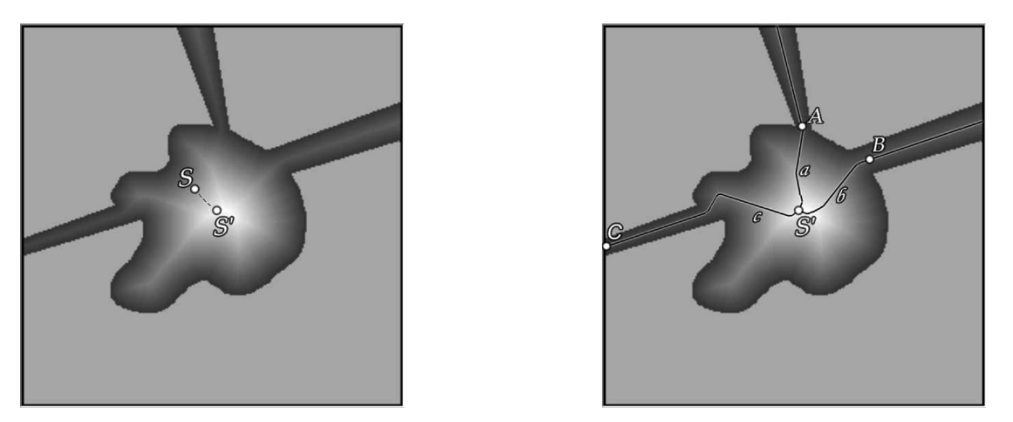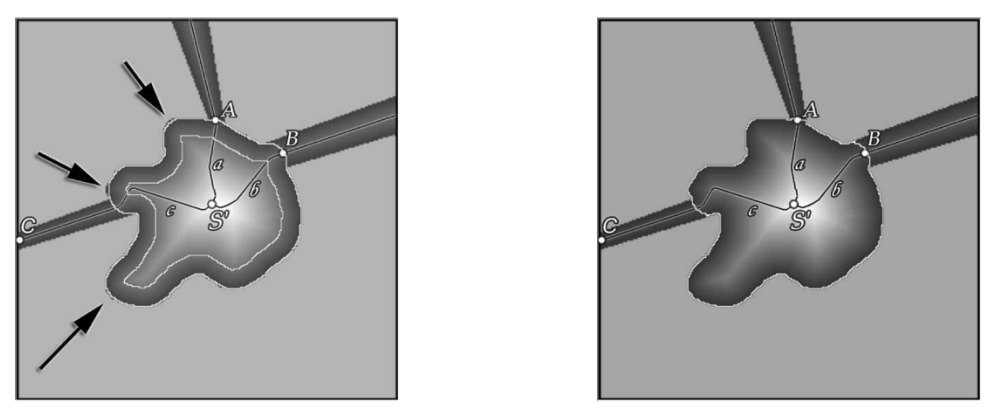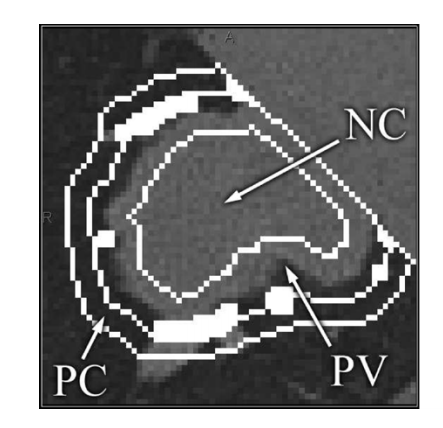# Morphological Segmentation and Partial Volume of Solid Pulmonary Lesions in Thoracic CT Scans

## 一 分割方法

### 1.1 描述

• P1):种子节点S位于结节内

• P2):结节完全位于VOI内部，并且没有触碰到VOI边缘

### 1.2 初始化### 1.3 从胸壁分离

CT图像中通常在结节与胸壁黏连之间并不表现出可见的强度对比。因此，此步骤仅重现使用形态学操作从胸壁分离的结节。

### 1.4 从黏连的脉管组织分离

1. 基本距离转换 ：为实现腐蚀和接下来的膨胀操作，使用两个相反的距离变换。首先是一个基本的3D欧几里得距离转换，从 $E:V\rightarrow R$ ,计算 $N_2$ 中每个体素到背景的最小距离。世界坐标用来计 算体素的各向异性：

$E(v):=min\lbrace\|\|world(v)-world(v')\|\|_2:v'\notin N_2\rbrace$ 接着在距离map中再使用低阈值 $threshold$ 来实现形态学腐蚀操作，称为腐蚀阈值。此操作的关键部分是找到合适的 $threshold$ ，在切除不想要的邻接结构时无须切除显著的结节边界特征。

2. 种子优化：为了优化初始种子节点S，目标病变区域的中心需要被近似。基本距离map E对 $N_2$ 中的每个体素到最邻近的体素的距离做了编码。从初始的定义的结节上的种子节点S出发，E上的局部最大搜索可以近似得到病变区域中心，如下图。• C1:大到足以切除所有靠近连接点的邻接结构

• C2: 与边界上限 $E_{\phi}(S’)$ 一致

1. 血管连接性模型：这些问题的答案取决于邻接结构和目标病变区域本身的形态学特征。我们为此提出了一个讨论框架，我们定义为血管连接性模型，对血管黏连做了如下两个假设：
• A1：每个肺组织最终源于肺门区域

• A2：每个组织的半径随着其到肺门距离的增加而单调递减。

1. 最优强度存在：由于我们期望算法能够区分非常规结节边界特征和邻接组织，我们需要利用先决条件P2:结节本身完全位于VOI内部并且没有触及其边界。由于假设A2，组织的半径在从肺门进入VOI之后不会增加。最终，它要么衰退为一个无法再追踪的度，要么再次离开了VOI。两种情况下，以特定腐蚀强度腐蚀的后果是，如果组织在某些点被腐蚀操作根除，此点之外的组织部分将会消失。

1. 最优腐蚀强度计算：下一步是高效的计算最优强度。利用 $E_{\phi}$ ，条件可以以最优种子节点S’到VOI边界的路径来表述。以 $P_{N_2}^{\gamma}$表示 $N_2$ 中任意长度 $n$ 的所有的路径集合 $(v_0,v_1,v_2,…v_n)$ ，从 $v_0=S’$ 开始在VOI边界上（基于3D邻居关系 $\gamma$）的体素 $v_n$ 终止。那么最优腐蚀强度 $\theta_{\star}$ 可以被定义为 :

1. 腐蚀：既然已经决定了合适的强度，在移除距离低于绝对腐蚀阈值 $\circleddash _:=\phi ^{-1}(\theta_)$的非归一化、基本距离mapE上可以做腐蚀操作。第一张图的图e显示了在距离map上使用阈值 $\circleddash _$之后的结果，结果得到的腐蚀过后的结节mask $N_$定义如下

$N\_:= \lbrace v\in N_2\|E(v)\ge \circleddash \_$ 注意到，此形态学操作通常无法成功将结节从胸壁上分离，由于肺连接通常更广，以及任意以必要强度完成的腐蚀操作要么会抹去任意非常规结节形态，要么移除整个结节。

1. 二次距离变换：从 $N_$ 开始，开运算的第二步，膨胀操作使用了一个二次距离变换map D，将每个体素映射到其到腐蚀结节mask $N _$ 的距离：## 二 基于分割的部分体积分析方法（SPVA）

SPVA分析定义如下：基于最终分析结果 $N_{\star}$ ，三个不同的区域，结节中心(nodule core NC)，薄壁组织区域(parenchyma area PC)，以及部分容积区域(partial volume PV)可以通过它们到最终mask $N_{\star}$ 边界的距离来自动确定。如下图